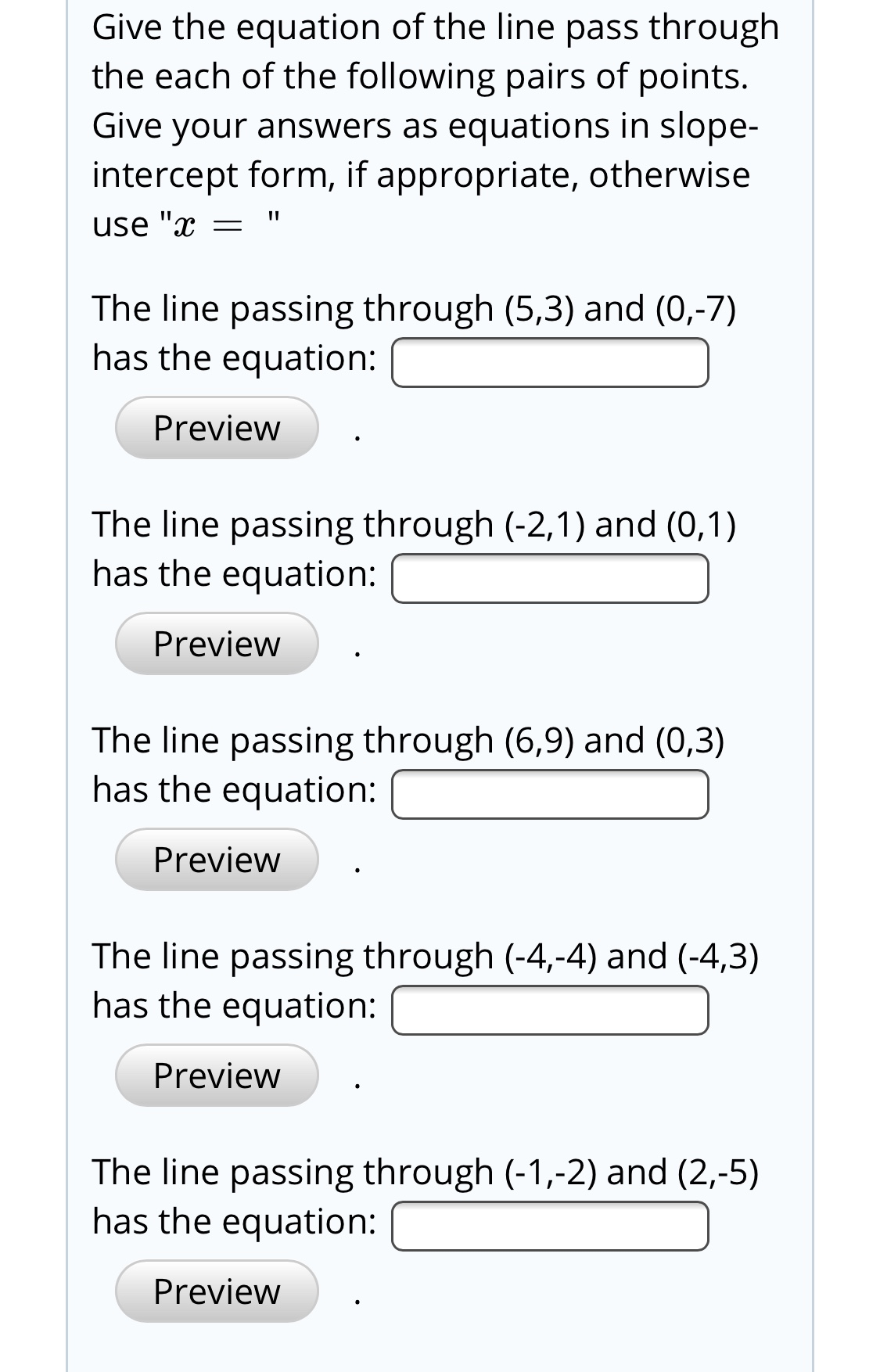# Give the equation of the line pass throughthe each of the following pairs of points.Give your answers as equations in slope-intercept form, if appropriate, otherwiseuse "xThe line passing through (5,3) and (0,-7)has the equation:PreviewThe line passing through (-2,1) and (0,1)has the equation:PreviewThe line passing through (6,9) and (0,3)has the equation:PreviewThe line passing through (-4,-4) and (-4,3)has the equation:PreviewThe line passing through (-1,-2) and (2,-5)has the equation:Preview

Question
6 viewshelp_outlineImage TranscriptioncloseGive the equation of the line pass through the each of the following pairs of points. Give your answers as equations in slope- intercept form, if appropriate, otherwise use "x The line passing through (5,3) and (0,-7) has the equation: Preview The line passing through (-2,1) and (0,1) has the equation: Preview The line passing through (6,9) and (0,3) has the equation: Preview The line passing through (-4,-4) and (-4,3) has the equation: Preview The line passing through (-1,-2) and (2,-5) has the equation: Preview fullscreen
check_circle

Step 1

Since you have asked multiple questions, we will solve the first question for you. If you want any specific question to be solved then please specify the question number or post only that question.

Step 2

Given:

Step 3

Calculation:

To find the slope intercept fo...

### Want to see the full answer?

See Solution

#### Want to see this answer and more?

Solutions are written by subject experts who are available 24/7. Questions are typically answered within 1 hour.*

See Solution
*Response times may vary by subject and question.
Tagged in